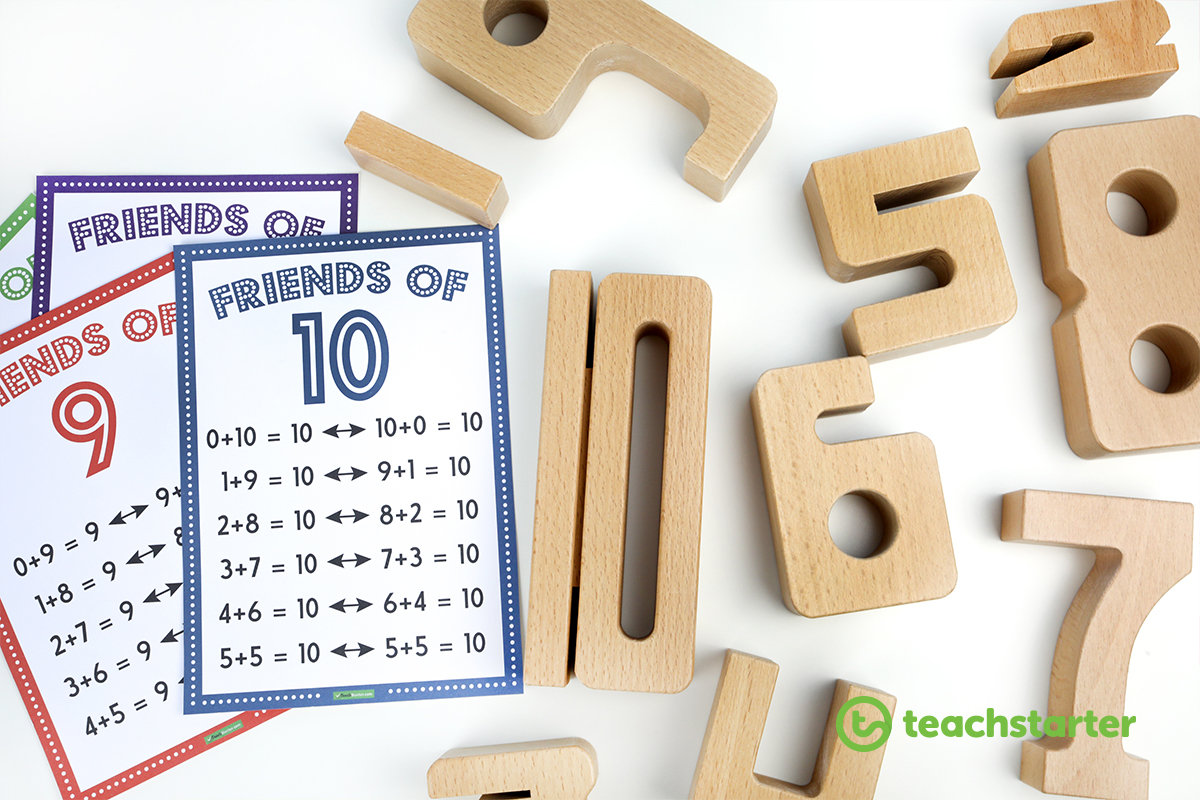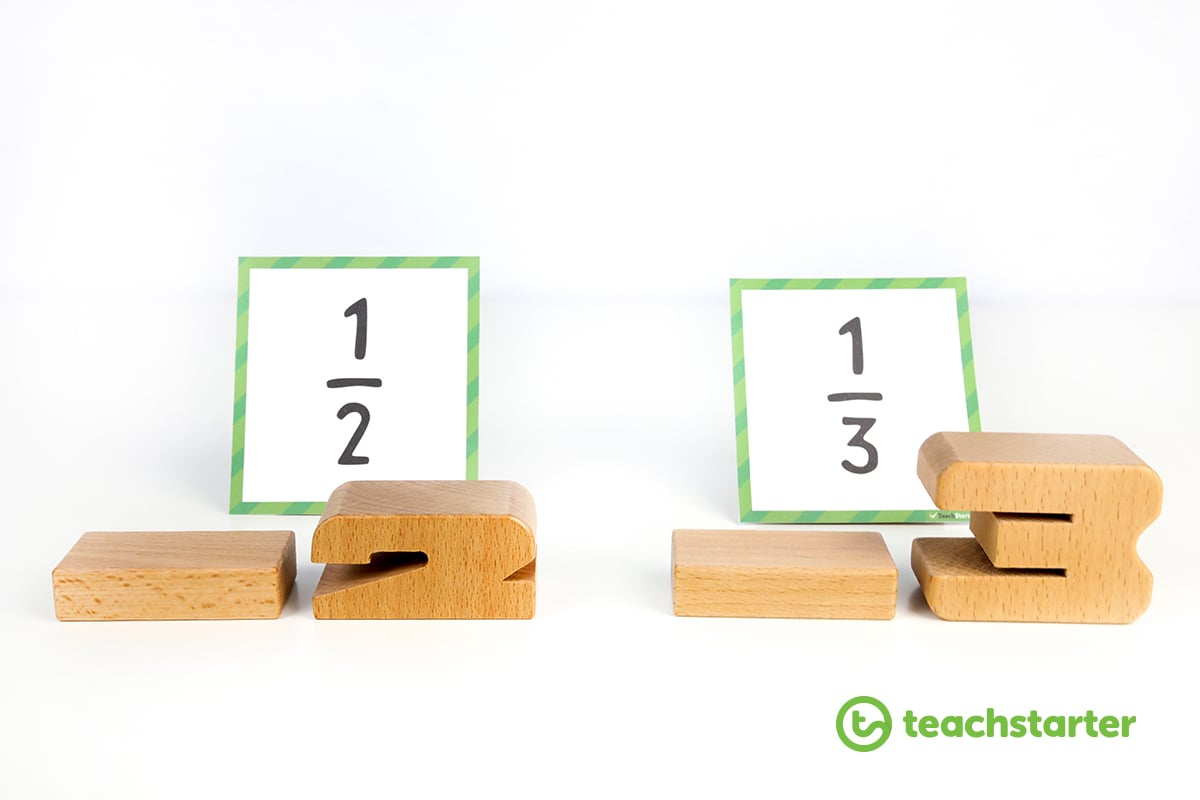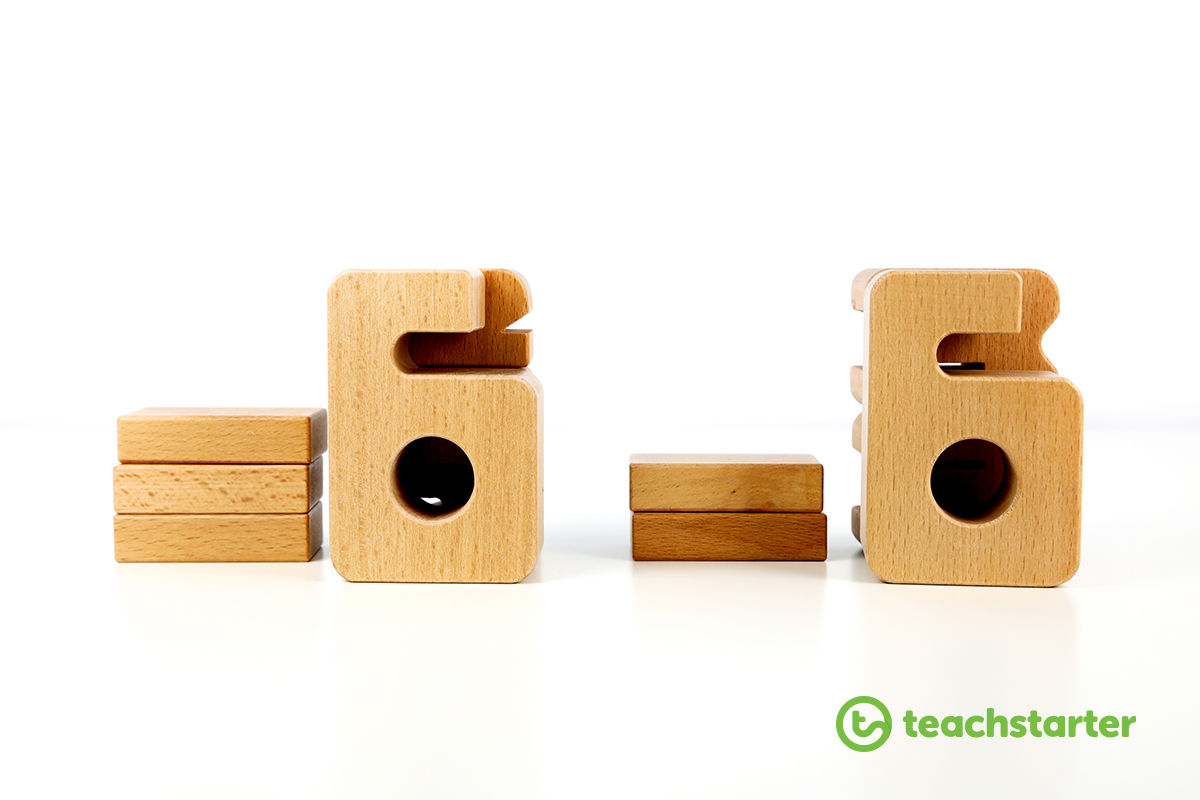Get more inspiration delivered to your inbox!

Teaching Ideas

# Sumblox - Academic Building Blocks Every Classroom Needs

Sumblox. These super clever wooden blocks are the next level of awesomeness… teaching the world of maths in the shape of wooden numbers.# What are Sumblox?

Sumblox are the latest mathematical tool on the market that empowers children of multiple ages to develop strong relationships with numbers. The height of the blocks corresponds to the value of the numbers. You can consolidate your students’ knowledge of addition, subtraction, multiplication, division and even fractions using this tactile visual resource.

Year 6 teachers are even using these blocks to teach fractions and algebra to their students. How cool is that?

# How Can I Use Sumblox in my Classroom?

We were lucky enough to get our hands on a box of these amazing blocks thanks to Biome. Check out some of the ways we thought you could use Sumblox in your classroom.

## Friends of Numbers

In the early years of mathematical understanding,  developing your students’ number sense can be tricky.

Repetition and varying the types of activities has always been one of the main ways you can help embed that knowledge. Teaching the relationship between different combinations of numbers and how they connect to make the same number is one skill that is crucial in the early years.

Enter Sumblox…

Because the height of the blocks correspond to the value of each of the numbers, the blocks create a great hands-on, visual understanding for students who are learning about ‘friends of…’ different numbers.

Using our Friends of… 1-10 Addition Posters, have students create the different combinations of numbers for each of the posters. A simple, yet effective hands-on activity that your students will be blown away with!

## Learning Multiplication

Understanding multiplication can be tricky for some students (I know I struggled at school). Sumblox can provide an additional way to visually represent multiplication.

Why not get students to represent different multiplication sums using Sumblox during your maths rotations in the classroom. For more ideas for setting up your maths rotations, head to our blog article – Ideas and Tips for Setting Up Maths Rotations in the Classroom.

Using our 0-12 Multiplication Flashcards. Students can turn over a sum and represent that sum using their Sumblox.

Who would have thought you could visually show the adding of fractions using blocks?

Using our Unit Fractions cards, students flip over two and are asked to add them together.

This is where Sumblox can provide that concrete material to help those tactile and visual learners.The students represent each fraction using the Sumblox.

They then need to make the denominator (second number) of each fraction the same height. However, they must only use that same number block.

Each time they add a block onto the denominator of each fraction, they must also add a number to the numerator (again, it must be the same number block).

In the example above, the students would need to add two ‘2’ blocks to the denominator and two ‘1’ blocks to the numerator.

For the second fraction, they would add one ‘3’ block to the denominator and one ‘1’ block to the numerator.

Re-creating the sum to 3/6 + 2/6. Easy peasy!All they need to do now is add the two numerators together, creating the answer of 5/6. Ta-dah!

If you are more a visual learner – we have created this awesome video to help explain our ideas!